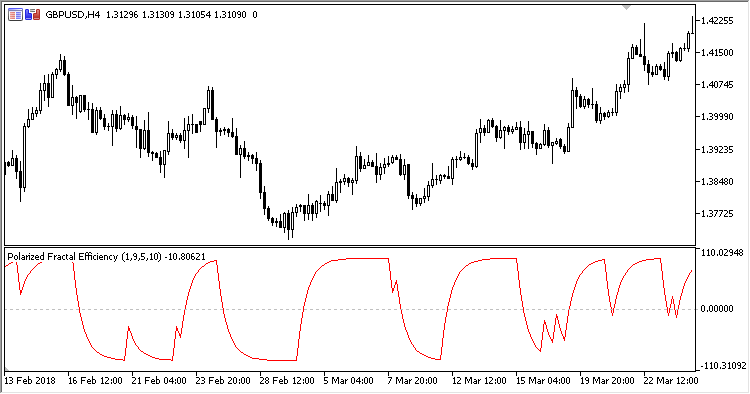Interesting script?
So post a link to it -
let others appraise it

You liked the script? Try it in the MetaTrader 5 terminal# PFE - indicator for MetaTrader 5

Views:
1106
Rating:
Published:
2018.09.27 15:03

The Polarized Fractal Efficiency (PFE) indicator is designed for identifying trends.

There are four input parameters:

• Fast ROC period - fast ROC (Rate of Change) calculation period;
• Slow ROC period - slow ROC (Rate of Change) calculation period;
• MA period - MA calculation period;
• PDS - oscillator scale factor.

Calculations:

`PFE = EMA(B, MA period)`

where:

• If Close > Close[Slow ROC period]:

`B = 100.0 * Z`
• Else:

`B = -100 * Z`
```Z = Sqrt(LongROC * LongROC + 100) / (Sqrt(ShortROC * ShortROC+1) + PDS)
LongROC = 100.0 * (Close / Close[Slow ROC period]-1)
ShortROC = 100.0 * (Close / Close[Fast ROC period]-1)
```

Interpretation:

• Oscillator value above zero - uptend;
• Oscillator value below zero - downtend.Translated from Russian by MetaQuotes Software Corp.
Original code: https://www.mql5.com/ru/code/21664Expert Ichimoku

An Expert Advisor based on the iIchimoku (Ichimoku Kinko Hyo) indicator.Exp_XWAMI_NN3_MMRec

Three independent trading systems using XWAMI indicators within a single EA with an ability to change the volume of a forthcoming trade depending on the results of the previous trades for this trading system.PFE_Overlay

The Polarized Fractal Efficiency Overlay (PFE_Overlay) indicator is designed for identifying trends. Unlike Polarized Fractal Efficiency, the indicator is displayed on the price chart as the main indicator line and two lines of the calculated StdDev deviation value.ROCX

ROCX is a modified ROC (Rate Of Change) indicator displaying an absolute or relative price change.